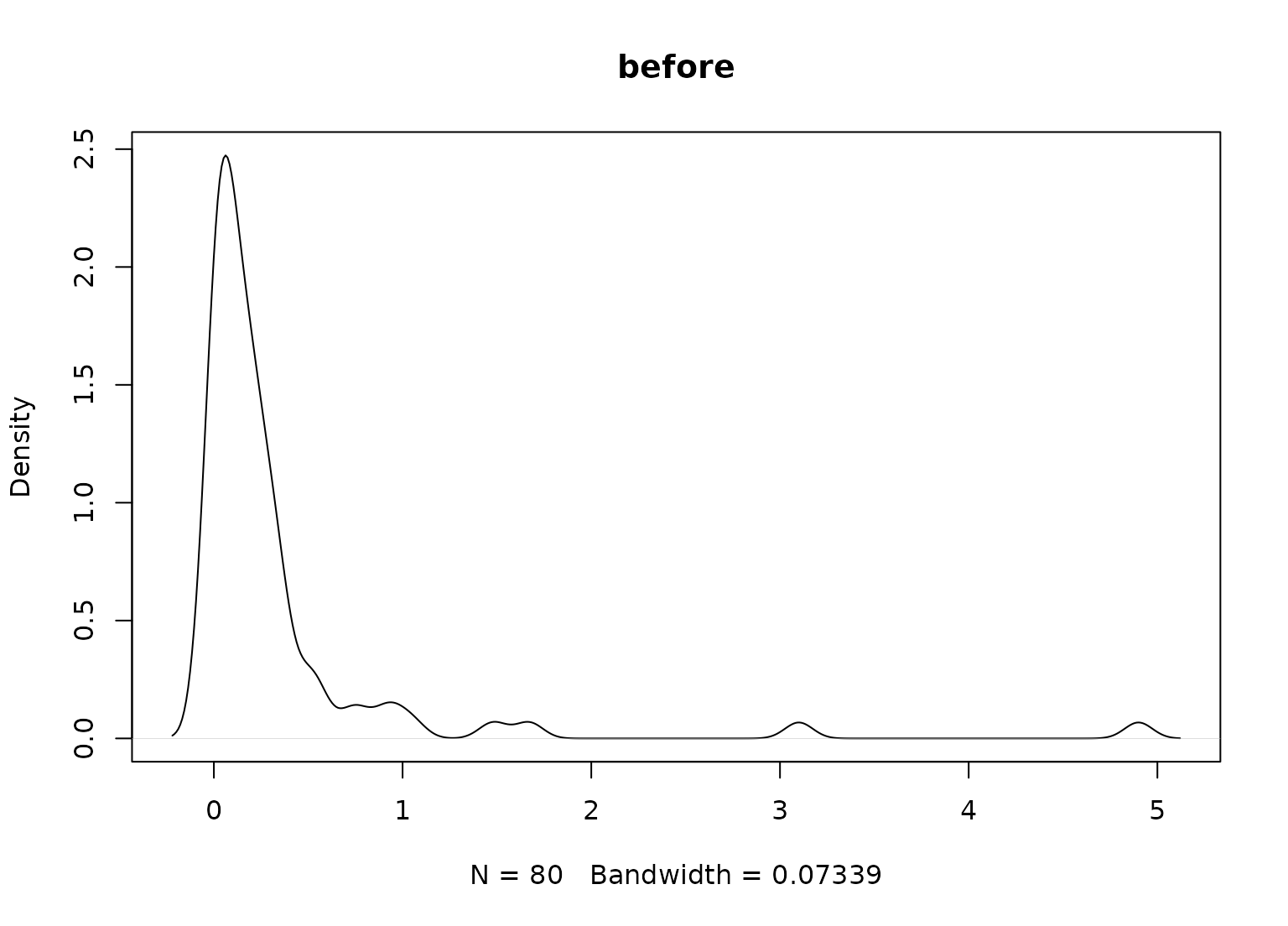step_YeoJohnson creates a specification of a recipe step that will transform data using a simple Yeo-Johnson transformation.

step_YeoJohnson(
recipe,
...,
role = NA,
trained = FALSE,
lambdas = NULL,
limits = c(-5, 5),
num_unique = 5,
na_rm = TRUE,
skip = FALSE,
id = rand_id("YeoJohnson")
)

## Arguments

recipe A recipe object. The step will be added to the sequence of operations for this recipe. One or more selector functions to choose variables for this step. See selections() for more details. Not used by this step since no new variables are created. A logical to indicate if the quantities for preprocessing have been estimated. A numeric vector of transformation values. This is NULL until computed by prep.recipe(). A length 2 numeric vector defining the range to compute the transformation parameter lambda. An integer where data that have less possible values will not be evaluated for a transformation. A logical value indicating whether NA values should be removed during computations. A logical. Should the step be skipped when the recipe is baked by bake.recipe()? While all operations are baked when prep.recipe() is run, some operations may not be able to be conducted on new data (e.g. processing the outcome variable(s)). Care should be taken when using skip = TRUE as it may affect the computations for subsequent operations. A character string that is unique to this step to identify it.

## Value

An updated version of recipe with the new step added to the sequence of any existing operations.

## Details

The Yeo-Johnson transformation is very similar to the Box-Cox but does not require the input variables to be strictly positive. In the package, the partial log-likelihood function is directly optimized within a reasonable set of transformation values (which can be changed by the user).

This transformation is typically done on the outcome variable using the residuals for a statistical model (such as ordinary least squares). Here, a simple null model (intercept only) is used to apply the transformation to the predictor variables individually. This can have the effect of making the variable distributions more symmetric.

If the transformation parameters are estimated to be very closed to the bounds, or if the optimization fails, a value of NA is used and no transformation is applied.

When you tidy() this step, a tibble with columns terms (the selectors or variables selected) and value (the lambda estimate) is returned.

Yeo, I. K., and Johnson, R. A. (2000). A new family of power transformations to improve normality or symmetry. Biometrika.

Other individual transformation steps: step_BoxCox(), step_bs(), step_harmonic(), step_hyperbolic(), step_inverse(), step_invlogit(), step_logit(), step_log(), step_mutate(), step_ns(), step_poly(), step_relu(), step_sqrt()

## Examples


library(modeldata)
data(biomass)

biomass_tr <- biomass[biomass$dataset == "Training",] biomass_te <- biomass[biomass$dataset == "Testing",]

rec <- recipe(HHV ~ carbon + hydrogen + oxygen + nitrogen + sulfur,
data = biomass_tr)

yj_transform <- step_YeoJohnson(rec,  all_numeric())

yj_estimates <- prep(yj_transform, training = biomass_tr)

yj_te <- bake(yj_estimates, biomass_te)

plot(density(biomass_te$sulfur), main = "before")plot(density(yj_te$sulfur), main = "after")tidy(yj_transform, number = 1)
#> # A tibble: 1 × 3
#>   terms         value id
#>   <chr>         <dbl> <chr>
#> 1 all_numeric()    NA YeoJohnson_s2EIn
tidy(yj_estimates, number = 1)
#> # A tibble: 6 × 3
#>   terms      value id
#>   <chr>      <dbl> <chr>
#> 1 carbon   -0.0225 YeoJohnson_s2EIn
#> 2 hydrogen  2.10   YeoJohnson_s2EIn
#> 3 oxygen    1.78   YeoJohnson_s2EIn
#> 4 nitrogen -0.830  YeoJohnson_s2EIn
#> 5 sulfur   -4.09   YeoJohnson_s2EIn
#> 6 HHV      -0.388  YeoJohnson_s2EIn